# A method of enhancing the Magnus effect by using blades instead of end plates

The Magnus effect is an efficient way to generate lift, which can be used in wind turbines, ship propulsion and aircraft. The traditional method of enhancing the Magnus effect is to add end plates to one or both ends of a cylindrical rotor, known as a Flettner rotor. This article proposes a new method(patented) to enhance the Magnus effect by replacing the end plates with a plurality of blades, which are briefly described as follows: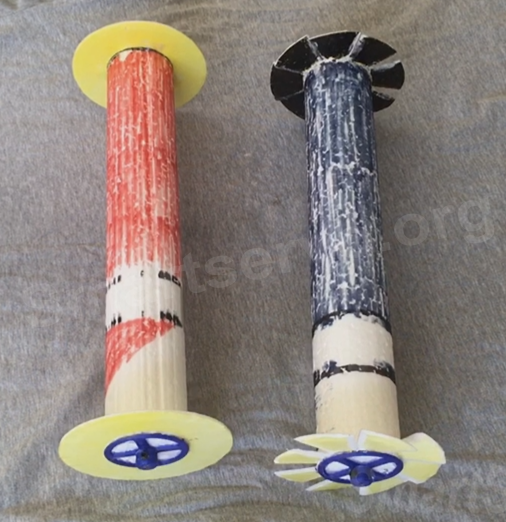On the left is a traditional rotor with end plates, on the right is a newly invented rotor with blades on the ends. The relevant patents are as follows:

• TWI 710501B
• CN 112141308B
• US 11143159B2

Characteristics of the newly invented rotor

The effect of the blades in the newly invented rotor is briefly described as.

1. When the blades act to exhaust the airflow from the end, the Magnus lift increases, the drag decreases, and the lift-to-drag ratio increases.
2. When the blades act to inhale the airflow from the end, the lift force decreases and the lift-drag ratio decreases.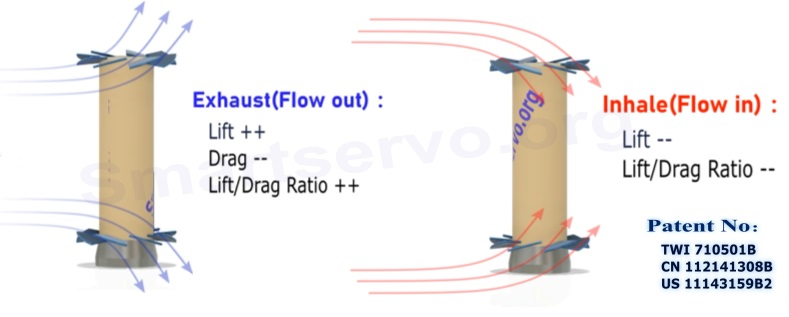A comparison experiment of this new rotor(blades) with the traditional rotor(end plates) is shown in the video below.

In this experiment, the diameter of the end plates of the traditional rotor is the same as the diameter of the blades of the new rotor. Furthermore, the area of all blades is equal to the area of all the end plates, so that when the pitches of the blades are set to 0, there is no difference in the aerodynamic characteristics of the two rotors. In other words, rotors using end plates can be regarded as a special case of rotors using blades.

Comparison of lift-to-drag ratios of the two rotors

The comparison diagrams of the two rotors is shown below, showing the relationship between lift-to-drag ratio and velocity ratio for different aspect ratios, respectively. while wind speed：2 m/sec，rotor diameter : 0.05 m，diameter of end plates/blades : 0.1 m, The velocity ratio refers to the ratio of rotor speed (rω) to wind speed.

• Aspect Ratio = 8（height：0.4 m, diameter：0.05 m）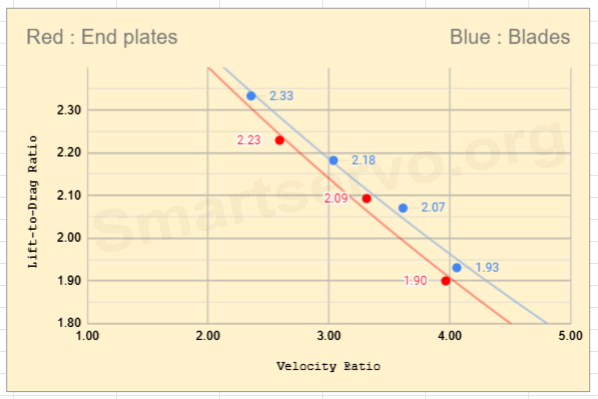• Aspect Ratio = 6（height：0.3 m, diameter：0.05 m）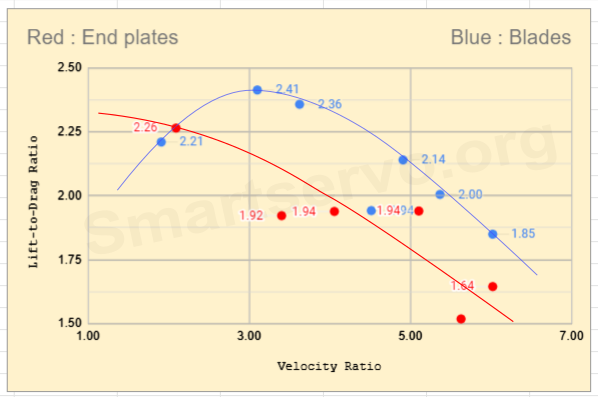• Aspect Ratio = 4（height：0.2 m, diameter：0.05 m）It can be seen from the above diagrams that the lift-to-drag ratio of the blue rotor(blades) is larger than that of the red rotor(end plates) when the velocity ratio is higher than a threshold, and the threshold is lower when the aspect ratio becomes smaller.

Comparison of lift force of the two rotors

The relationship between the lift (gw) and the velocity ratio of the two rotors for different aspect ratios is as follows, the wind speed：2 m/sec, pitch angle of blades ≈10°, The velocity ratio refers to the ratio of rotor speed (rω) to wind speed.

• Aspect Ratio = 8（height：0.4 m, diameter：0.05 m）, diameter of end plates/blades = 0.1 m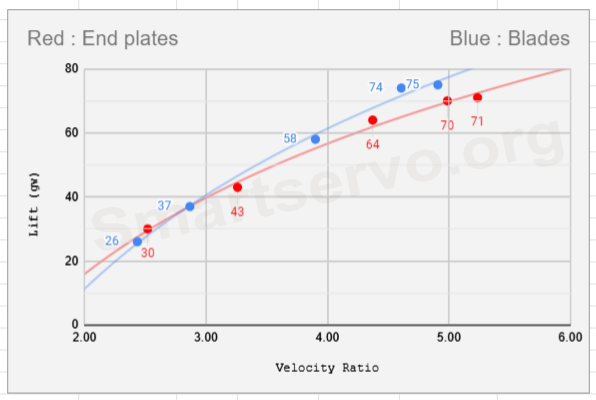• Aspect Ratio = 5（height：0.4 m, diameter：0.08 m）, diameter of end plates/blades = 0.13 m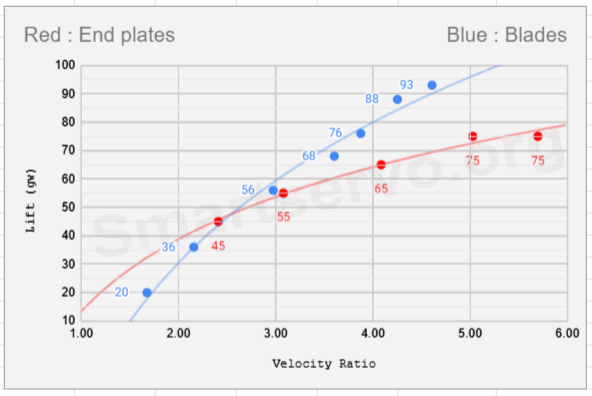• Aspect Ratio = 1.5（height：0.3 m, diameter：0.2 m）, diameter of end plates/blades = 0.27 m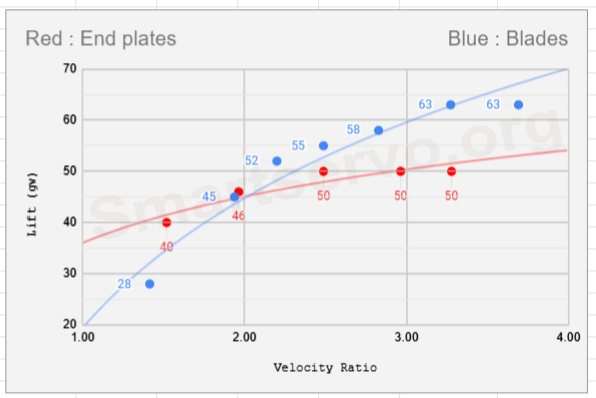The diagrams above show that the lift generated by the blue rotor(blades) is larger than that of the red rotor(end plates) when the velocity ratio is higher than a threshold, and the threshold is lower when the aspect ratio becomes smaller.